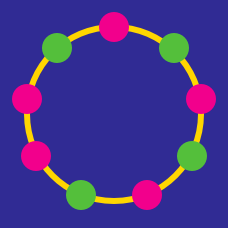Probability

# Recursion Warmup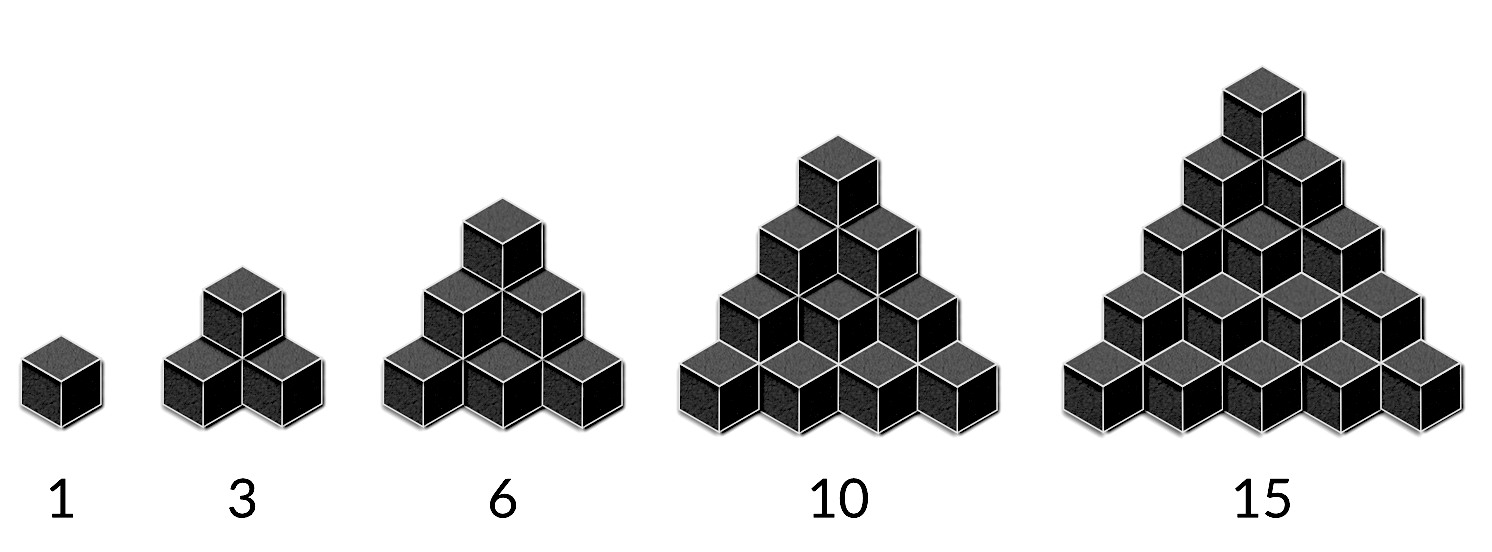What is the next number in this sequence? (There are no hidden cubes.)

$0, 1, 3, 6, 10, 15...$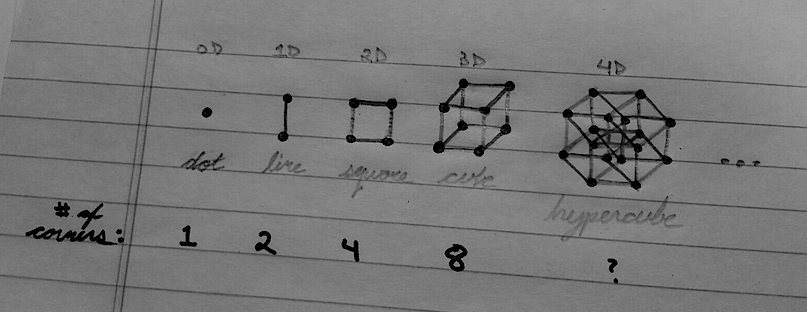How many corners does a hypercube (a 4-dimensional cube) have?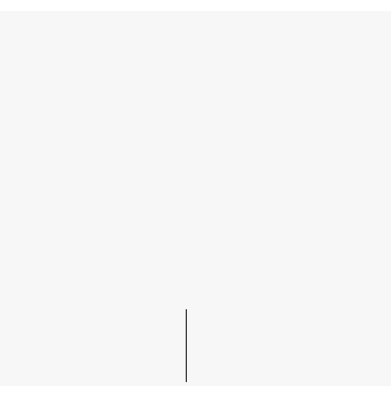How many line-segments are there in the 4th image of this growing tree sequence?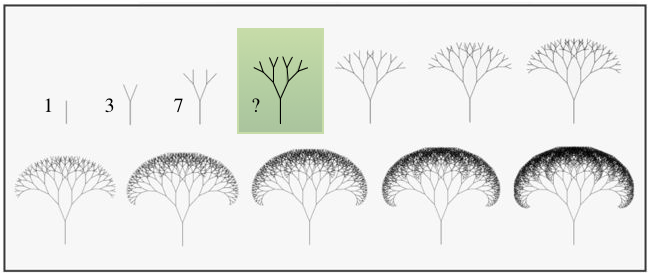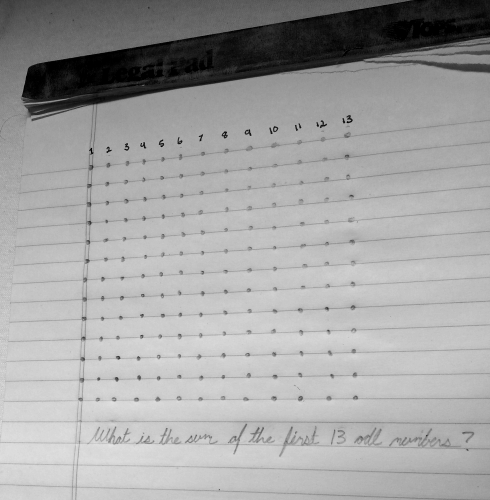What is the sum of the first 13 positive odd numbers?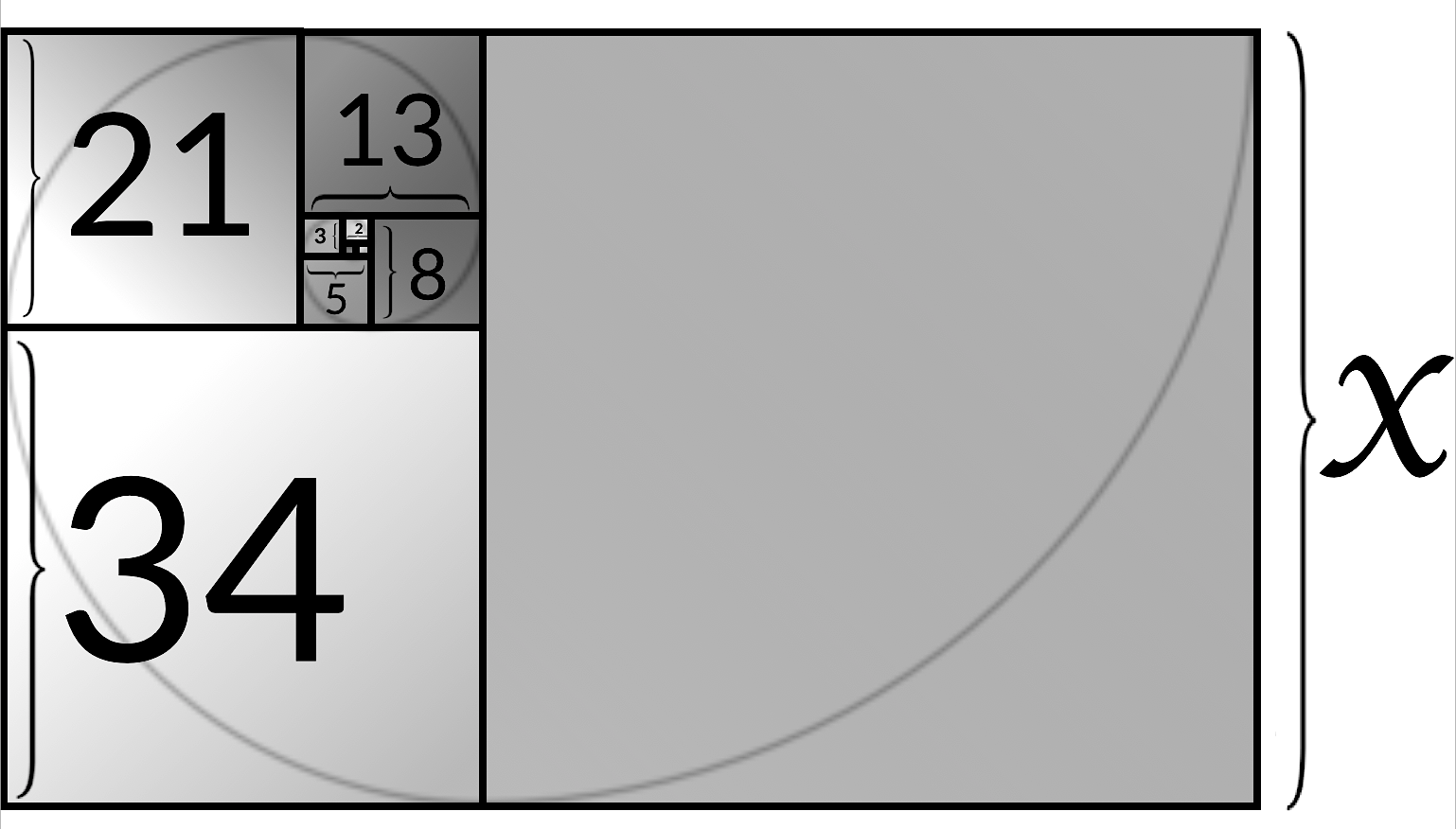The sizes of the first 9 squares that make this spiral are:

$$1, 1, 2, 3, 5, 8, 13, 21, 34...$$

How big is the 10th square added to the spiral?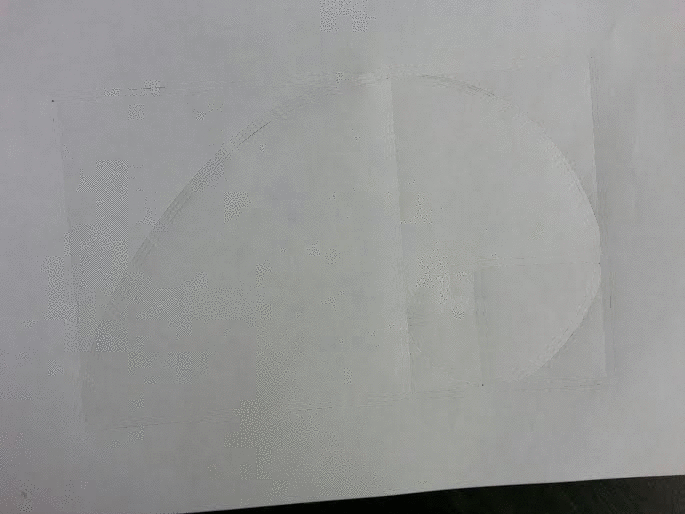×